microprocessors. A microprocessor is an integrated circuit designed for two purposes: data processing and control. Computers and microprocessors both operate on a series of electrical pulses called words. A word can be represented by a binary number such as 101100112. The word length is described by the number of digits or BITS in the series. A series of four digits would be called a 4-bit word and so forth. ">Custom SearchBINARY-CODED DECIMAL In today's technology, you hear a great deal about microprocessors. A microprocessor is an integrated circuit designed for two purposes: data processing and control. Computers and microprocessors both operate on a series of electrical pulses called words. A word can be represented by a binary number such as 101100112. The word length is described by the number of digits or BITS in the series. A series of four digits would be called a 4-bit word and so forth. The most common are 4-, 8-, and 16-bit words. Quite often, these words must use binary-coded decimal inputs. Binary-coded decimal, or BCD, is a method of using binary digits to represent the decimal digits 0 through 9. A decimal digit is represented by four binary digits, as shown below:You should note in the table above that the BCD coding is the binary equivalent of the decimal digit. Since many devices use BCD, knowing how to handle this system is important. You must realize that BCD and binary are not the same. For example, 4910 in binary is 1100012, but 4910 in BCD is 01001001BCD. Each decimal digit is converted to its binary equivalent. BCD Conversion You can see by the above table, conversion of decimal to BCD or BCD to decimal is similar to the conversion of hexadecimal to binary and vice versa. For example, let's go through the conversion of 26410 to BCD. We'll use the block format that you used in earlier conversions. First, write out the decimal number to be converted; then, below each digit write the BCD equivalent of that digit: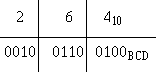The BCD equivalent of 26410 is 001001100100BCD. To convert from BCD to decimal, simply reverse the process as shown: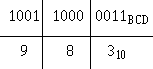BCD Addition The procedures followed in adding BCD are the same as those used in binary. There is, however, the possibility that addition of BCD values will result in invalid totals. The following example shows this: Add 9 and 6 in BCD: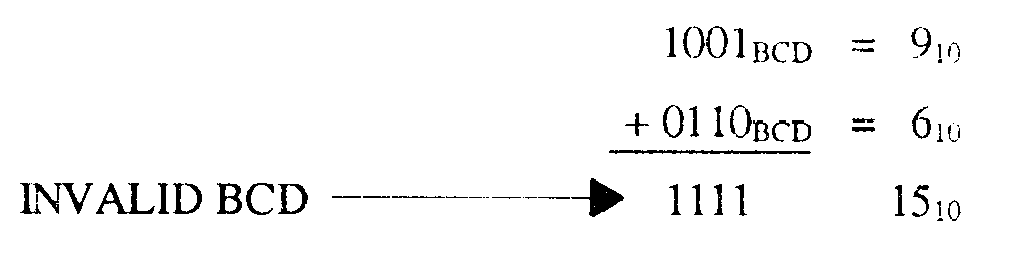The sum 11112 is the binary equivalent of 1510; however, 1111 is not a valid BCD number. You cannot exceed 1001 in BCD, so a correction factor must be made. To do this, you add 610 (0110BCD) to the sum of the two numbers. The "add 6" correction factor is added to any BCD group larger than 10012. Remember, there is no 10102, 10112, 11002, 11012, 11102, or 11112 in BCD: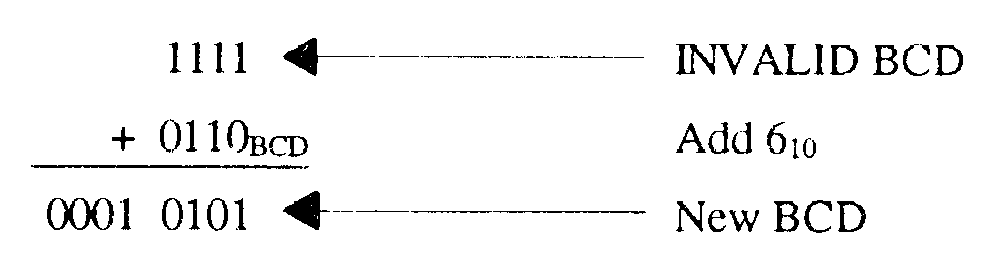The sum plus the add 6 correction factor can then be converted back to decimal to check the answer. Put any carries that were developed in the add 6 process into a new 4-bit word: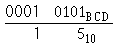Now observe the addition of 6010 and 5510 in BCD: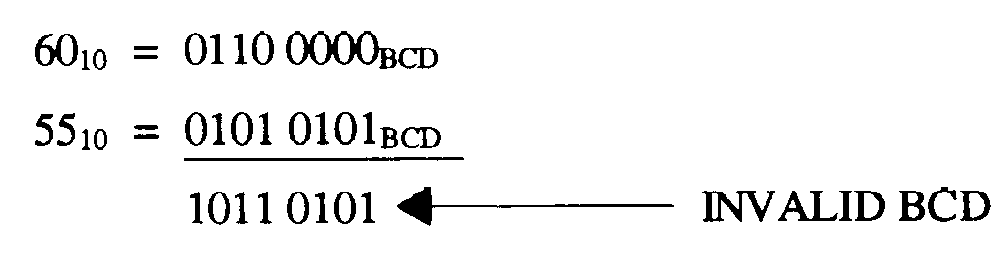In this case, the higher order group is invalid, but the lower order group is valid. Therefore, the correction factor is added only to the higher order group as shown: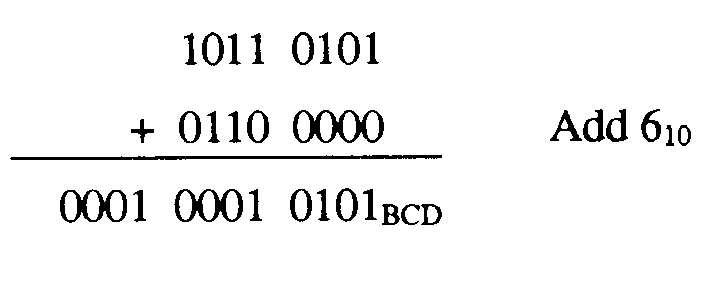Convert this total to decimal to check your answer: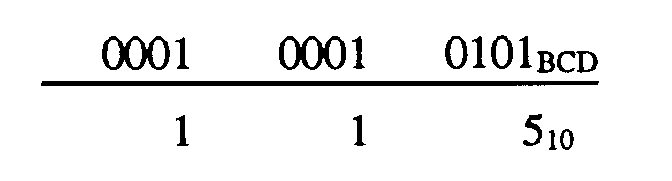Remember that the correction factor is added only to groups that exceed 910 (1001BCD). Convert the following numbers to BCD and add: Q107.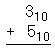Q108.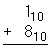Q109.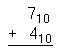Q110.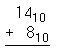Integrated Publishing, Inc. - A (SDVOSB) Service Disabled Veteran Owned Small Business